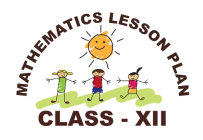### Resource Centre Mathematics

Resource Centre Mathematics Mathematics worksheet, mathematics basic points and formulas, mathematics lesson plan, mathematics multiple choice questions Workplace Dashboard CBSE Syllabus For Session 2023-24 For  :   Classes IX & X    |   Classes XI & XII Watch Videos on Maths Solutions CLASS IX MATHEMATICS FORMULAS &  BASIC CONCEPTS

### Lesson Plan Math Class 12 (Ch-3) | Matrices

E- LESSON PLAN   SUBJECT MATHEMATICS    CLASS 10+2
Lesson Plan Class 12th chapter 3 Matrices Subject Mathematics for Mathematics Teacher. Effective way of Teaching Mathematics. Top planning by the teacher for effective teaching in the class.Board – CBSE CLASS –XII SUBJECT- MATHEMATICS CHAPTER 3  :-  Matrices

TOPIC:- Chapter : 3 : Matrices

DURATION:-

This chapter is divided into eight modules and is completed in 15 class meetings.

PRE- REQUISITE KNOWLEDGE:-

Basic knowledge of algebra, and some simple functions.

TEACHING AIDS:-
Green Board, Chalk,  Duster, Charts, smart board, projector, laptop etc.

METHODOLOGY:-   Lecture method

OBJECTIVES:-
• Introduction and concepts of matrices with different definitions, addition, subtraction, multiplication of two matrices and multiplication of matrices with a scalar.
• Transpose of the matrices.
• Symmetric and skew symmetric matrices.
• Concept of elementary row and column operations.
• Method of finding the inverse of the matrices if exist by using elementary operations.
PROCEDURE :-
Start the session by asking some questions about the previous knowledge of the students and by introducing the concept of matrices in general form. Now introduce the different topics step by step as follows.

 S.N Topic 1 Introduction of matrices, order of the matrices, elements of matrices, definitions of zero, identity, square and diagonal matrices. 2 Operations on the matrices addition, subtraction, multiplication and multiplication of matrices with scalar. 3 Transpose of the matrices and its properties, Symmetric and skew symmetric matrices. 4 Representation of square matrices as the sum of symmetric and skew symmetric matrix. 5 Existence of non-zero matrices (square matrices of order 2) whose product is zero. 6 Concept of elementary row and column operations. 7 Invertible matrices and proof of uniqueness of inverse, if it exists. 8 Method of finding the inverse of the square matrices by using elementary operations.

EXPECTED OUTCOMES:-
After studying this lesson students should know
• different types of matrices, their addition, subtraction and multiplication.
• Students should know the transpose of the matrix and are able to write the given matrix as the sum of symmetric and skew symmetric matrices.
• Students should be able to find the inverse of matrices by applying elementary row or column operations.
STUDENTS DELIVERABLES:-
• Review questions given by the teacher.
• Solve NCERT problems with examples, solve assignment given by the teacher.
EXTENDED LEARNING:-
Students can extend their learning in Mathematics through the RESOURCE CENTRE.  Students can also find many interesting topics on mathematics at cbsemathematics.com

ASSESSMENT TECHNIQUES:-
• Assignment sheet will be given as home work at the end of the topic.
• Separate sheets which will include questions of logical thinking and Higher order thinking skills will be given to the above average students.
• Class Test , Oral Test , worksheet and Assignments. can be made the part of assessment.
• Re-test(s) will be conducted on the basis of the performance of the students in the test.

🙏

1.